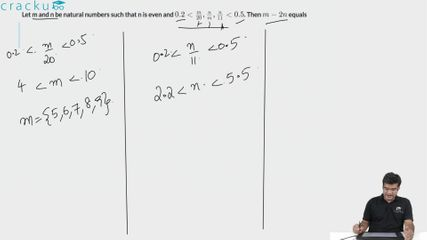Question 59

# Let m and n be natural numbers such that n is even and $$0.2<\frac{m}{20},\frac{n}{m},\frac{n}{11}<0.5$$. Then $$m-2n$$ equals

Solution

$$0.2<\frac{n}{11}<0.5$$

=> 2.2<n<5.5

Since n is an even natural number, the value of n = 4

$$0.2<\frac{m}{20}<0.5$$  => 4< m<10. Possible values of m = 5,6,7,8,9

Since $$0.2<\frac{n}{m}<0.5$$, the only possible value of m is 9

Hence m-2n = 9-8 = 1

### View Video Solution